To Top

## 1. 简介

T2T 能够帮助人们为各种机器学习程序创建最先进的模型，可应用于多个领域，如翻译、语法分析、图像信息描述等，大大提高了研究和开发的速度。T2T 中也包含一个数据集和模型库，其中包括谷歌近期发布的几篇论文中提出的最新模型（Attention Is All You Need、Depthwise Separable Convolutions for Neural Machine Translation 和 One Model to Learn Them All）。

T2T 库中的两个模型：SliceNet 和 Transformer 的表现超过了此前的业界最佳水平 GNMT+MoE。其中，Transformer 的分数超过 GNMT 模型 3.8 分，而 GNMT 已经超过基准水平（基于短语的翻译系统 MOSES）4 分了。值得注意的是，使用 T2T，你可以用一块 GPU，一天的时间实现此前业界最佳水准的表现：使用小型 Transformer 模型，在单 GPU 训练一天时间后可以达到 24.9 的 BLEU 分数。现在，所有人都可以自己构建最好的翻译模型了。

## 2. 模块化多任务训练

T2T 库构建于 TensorFlow 之上，定义了深度学习系统所需的各个组件：数据集、模型架构、优化器、学习速率衰减方案、超参数等等。最重要的是，它实现了所有这些组件之间的标准对接形式，并使用了目前最好的机器学习方法。这样，你可以选择任何一组数据集、模型、优化器，然后设定超参数，开始训练，并查看它的性能。通过架构的模块化，在输入和输出数据之间的每一块都是张量-张量的函数。这意味着如果你对模型架构有了新想法，你无须改动整个模型，你可以保留嵌入部分、损失函数和其他所有部分，仅仅用自己的函数替换模型体的一部分，该函数将张量作为输入，并返回一个张量。

## 4. Attention Is All You Need

### 4.1 背景

rnn需要当前时间步的状态，以及之前时间步的状态，所以这种序列特性天生地（inherently）难以并行，当序列较长时，由于内存有限，这缺陷尤为明显。最近的解决方法主要有两种：factorization tricks《Factorization tricks for LSTM networks》和conditional computation《Outrageously large neural networks: The sparsely-gated mixture-of-experts layer》[Conditional computation, where parts of the network are active on a per-example basis, has been proposed in theory as a way of dramatically increasing model capacity without a proportional increase in computation.]。但序列计算的局限性一直还在。

Extended Neural GPU(《Can active memory replace attention?》)、ByteNet(《Neural machine translation in linear time》)、ConvS2S（《Convolutional Sequence to Sequence Learning》）都使用CNN并行地计算所有输入和输出位置的隐层表示，从而减少序列计算。其中，对任意两个输入和输出位置进行计算与他们间的距离有关，ConvS2S是线性时间复杂度，ByteNet是log时间复杂度。而Transformer则是常数时间复杂度(前提是the cost of reduced effective resolution due to averaging attention-weighted positions, an effect we counteract with Multi-Head Attention)。

### 4.2 架构

#### 4.2.1 encoder and decoder stacks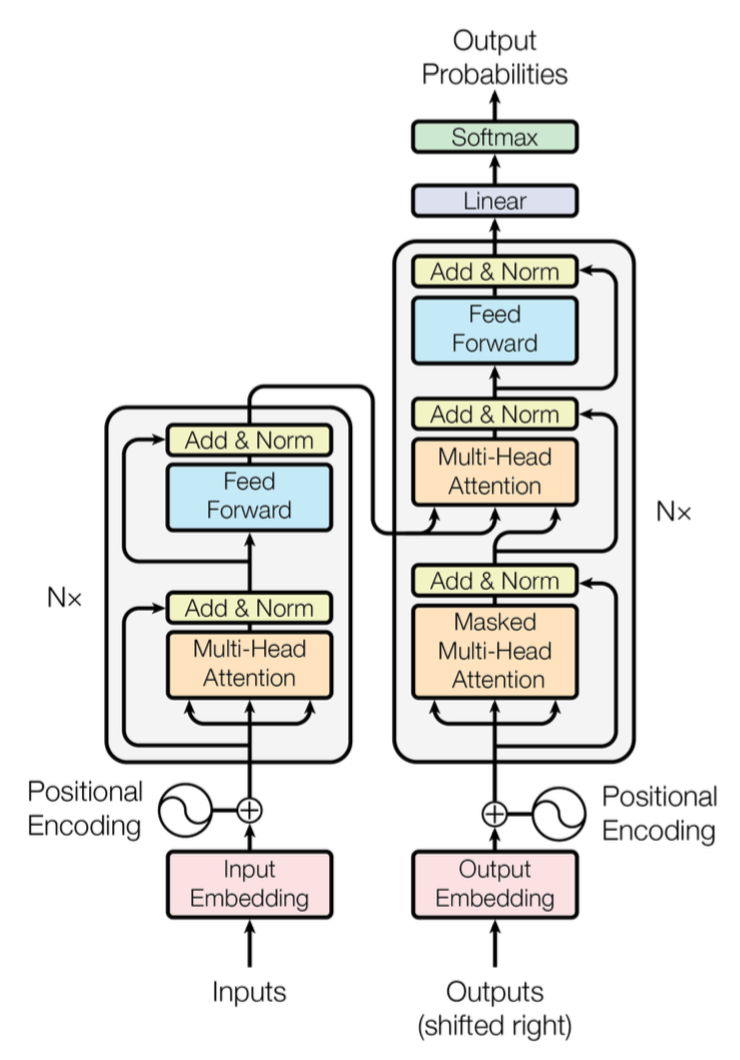• encoder:

encoder堆叠了N=6层，每层有两个子层：

• position-wise的全连接层

• decoder:

#### 4.2.2 attention

attention函数：将一个query和一系列的(key, value)对映射起来。其中，query、key、value均是向量，维度分别为$$d_k$$, $$d_k$$, $$d_v$$最终的输出是各value的加权组合（v的权重w其实就是下面公式里V的系数$$softmax(\frac{QK^T}{\sqrt{d_k}})$$）：

$q->[(k_1,v_1), (k_2,v_2), ...]->attention=w_1*v_1+w_2*v_2+...$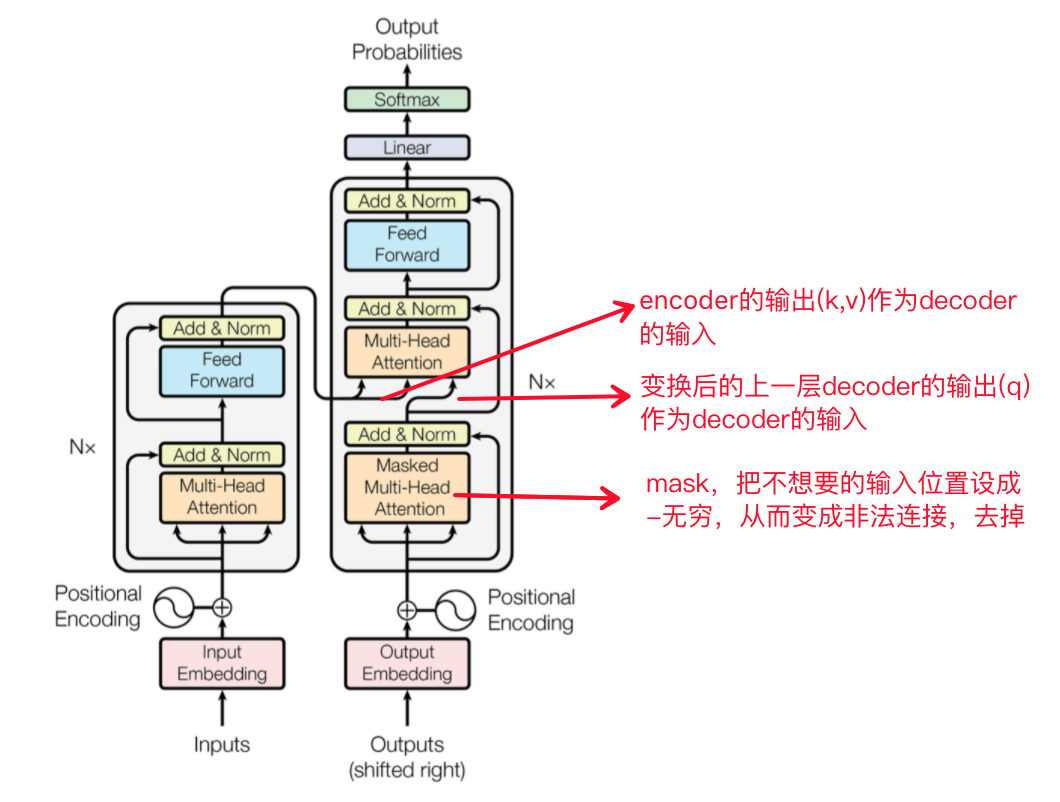• scaled dot-product attention

query和所有key算点积，然后除以$$\sqrt{d_k}$$，然后算softmax，得到的就是value的weight。实际应用中，一系列的query，记为矩阵$$Q$$，同样地，有$$K$$$$V$$

$Attention(Q,K,V)=softmax(\frac{QK^T}{\sqrt{d_k}})V$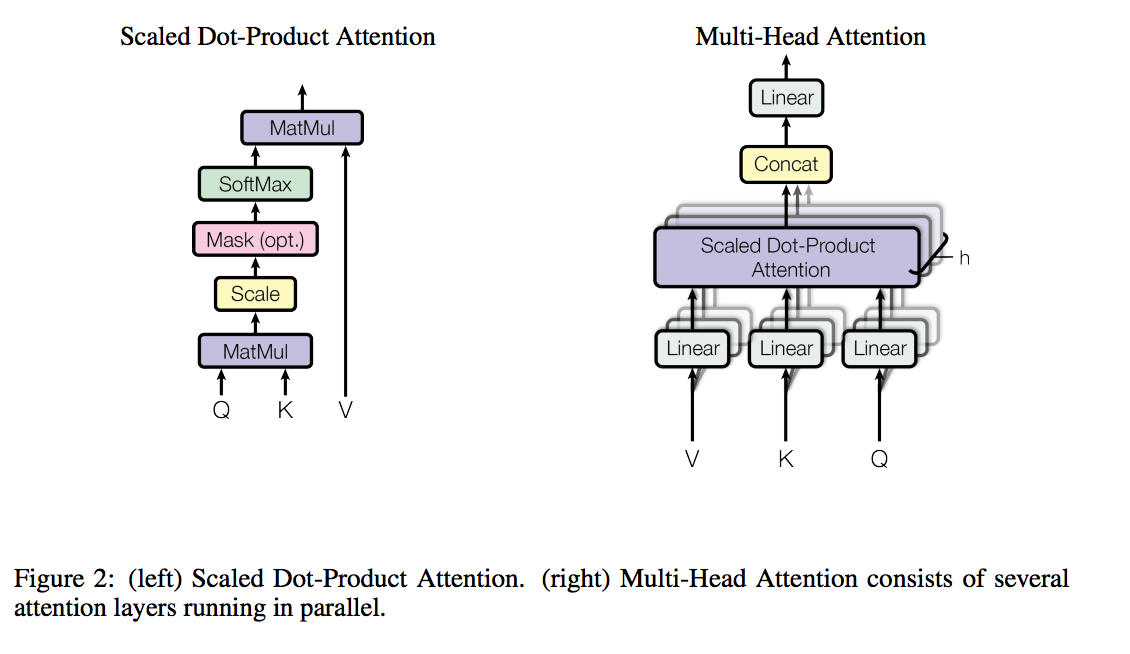$$d_k$$不大时，additive attention比没用scale的dot-product attention表现更好。但$$d_k$$很大时，点积会变得特别大，所以softmax会出现有些区域的梯度变得特别小（例如，假设$$q$$$$k$$是独立的随机变量，均值都是0，方差都是1，那么他们的点积$$qk=\sum^{d_k}_{i=1}q_ik_i$$的均值是0，方差是$$d_k*1^2=d_k$$。【详见下面的两个随机变量的和与积的方差推导】），因此，需要通过除以$$\sqrt{d_k}$$来scale。

$\\设 X 与 Y 是两个随机变量，则方差 \\V(X+Y)= V(X)+V(Y)+2Cov(X,Y) \\V(X -Y)= V(X)+V(Y)-2Cov(X,Y) \\特别的，当X，Y是两个不相关的随机变量则 \\V(X+Y)=V(X)+V(Y),V(X-Y)=V(X)+V(Y)$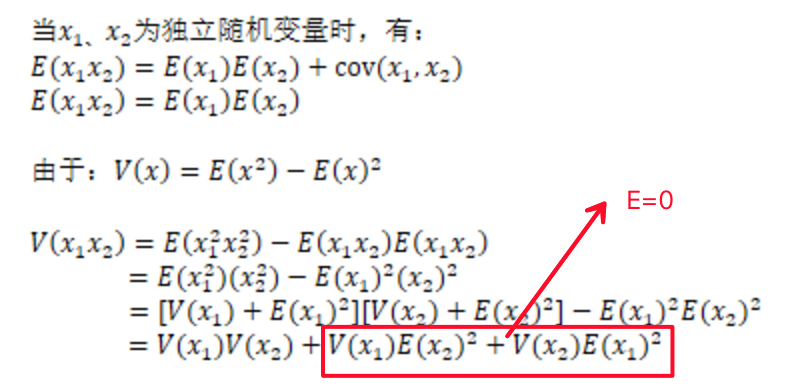$\\MultiHead(Q,K,V)=Concat(head_1,...,head_h)W^O \\where\ head_i=Attention(QW_i^Q,KW_i^K,VW_i^V)$

• $$W_i^Q\in \mathbb{R}^{d_{model}\times d_k}$$
• $$W_i^K\in \mathbb{R}^{d_{model}\times d_k}$$
• $$W_i^V\in \mathbb{R}^{d_{model}\times d_v}$$
• $$W^O\in \mathbb{R}^{hd_v\times d_{model}}$$

• encoder-decoder结构中，对当前层的decoder而言，query来自前一层的decoder，key和value来自encoder的输出。
• encoder有self-attention层。在self-attention中，所有层的key，value和query都来自于前一层encoder的输出。因此，当前层的encoder的每个位置可以attend to前一层的所有位置。

#### 4.2.3 position-wise feed-forward networks

$FFN(x)=ReLU(xW_1+b_1)W_2+b_2=max(0, xW_1+b_1)W_2+b_2$

#### 4.2.5 positional encoding

$\\PE_{(pos,2i)}=sin(pos/10000^{2i/d_{model}}) \\PE_{(pos,2i+1)}=cos(pos/10000^{2i/d_{model}})$

pos是位置，i是维度。因为对于任意固定的offset k，$$PE_{pos+k}$$能被表示为$$PE_{pos}$$的线性函数。即把id为$$pos$$的位置，映射成一个$$d_{model}$$维的向量，这个向量的第i个元素的数值是$$PE_{(pos,i)}$$

• 以前在RNN、CNN模型中其实都出现过Position Embedding，但在那些模型中，Position Embedding是锦上添花的辅助手段，也就是“有它会更好、没它也就差一点点”的情况，因为RNN、CNN本身就能捕捉到位置信息。但是在这个纯Attention模型中，Position Embedding是位置信息的唯一来源，因此它是模型的核心成分之一，并非仅仅是简单的辅助手段。
• Position Embedding本身是一个绝对位置的信息，但在语言中，相对位置也很重要，Google选择前述的位置向量公式的一个重要原因是：有$$\sin(\alpha+\beta)=\sin\alpha\cos\beta+\cos\alpha\sin\beta$$，也有$$\cos(\alpha+\beta)=\cos\alpha\cos\beta-\sin\alpha\sin\beta$$，所以，位置p+k的向量可以表示成位置p的向量的线性变换，这提供了表达相对位置信息的可能性。例如，假设p是奇数(它的pe是cosp)，k是偶数(它的pe是sink)，那p+k是奇数，所以他的pe是$$cos(p+k)=cosp\cdot cosk-sinp\cdot sink=C_1\cdot cosp-C_2\cdot sink$$，是p和k的pe的各自的线性变换。类似的p是奇数，k是奇数，p+k是偶数,$$sin(p+k)=sinp\cdot cosk+cosp\cdot sink=C_1\cdot cosk+C_2\cdot cosp$$

#### 4.2.6 why self-attention

1. 每一层的总的计算复杂度
2. 能被并行化的计算量（可以用”所需要的最小的序列操作“来衡量）
3. 网络中长距离依赖的路径长度

• 首先，对self-attention与recurrrent进行对比：

self-attention需要的序列操作是常数级别的，而recurrent需要$$O(n)$$

• 然后，看convolutional

• self-attention还有一个好处，产出的模型更加可解释（interpretable）。Layer Type Complexity per Layer Sequential Operations Maximum Path Length
Self-Attention $$O(n^2\times d)$$ $$O(1)$$ $$O(1)$$
Recurrent $$O(n\times d^2)$$ $$O(n)$$ $$O(n)$$
Convolutional $$O(k\times n\times d^2)$$ $$O(1)$$ $$O(log_k(n))$$
Self-Attention(restricted) $$O(r\times n\times d)$$ $$O(1)$$ $$O(n/r)$$

#### 4.2.7 生成过程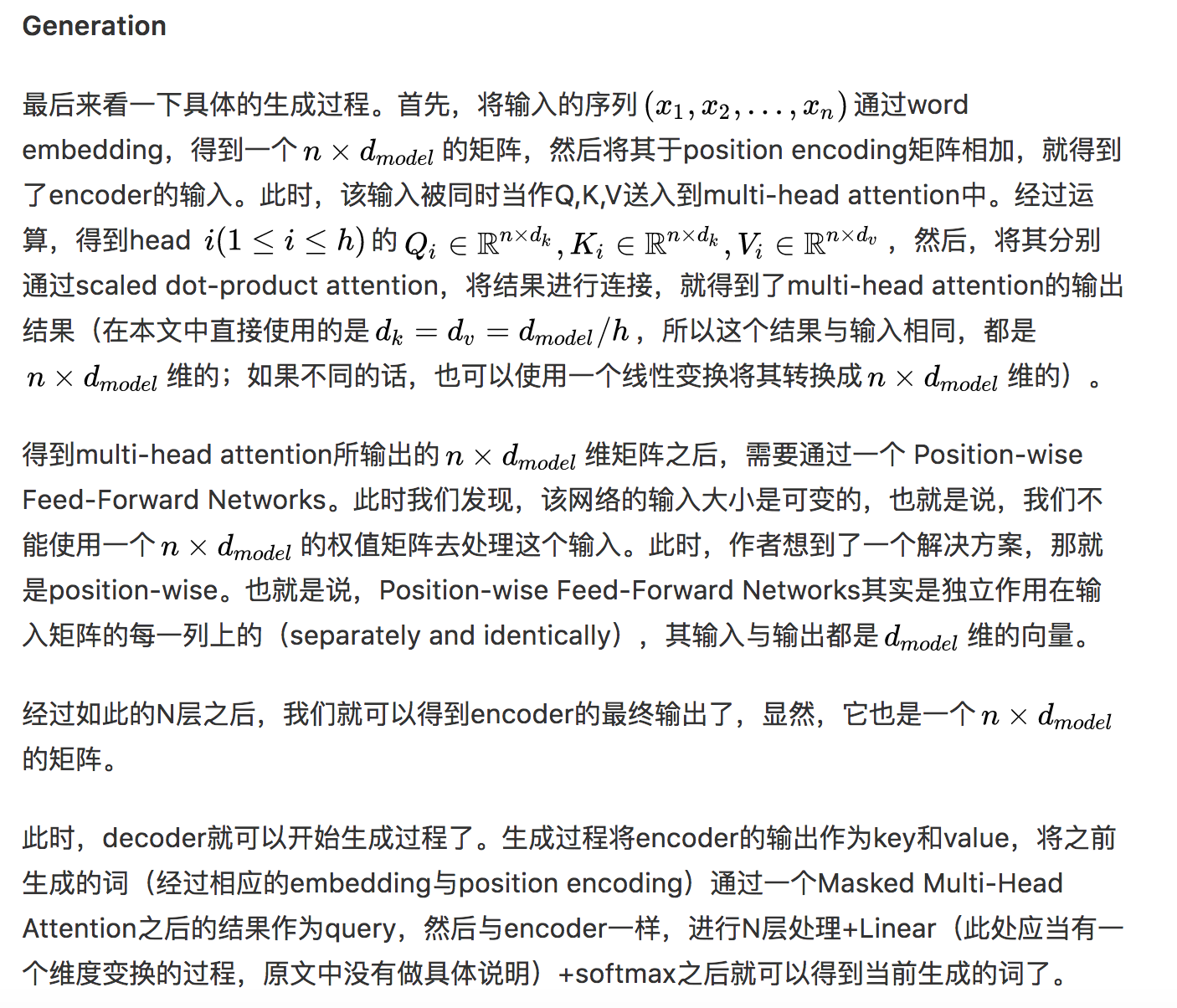### 4.4 结果

#### 4.4.1 机器翻译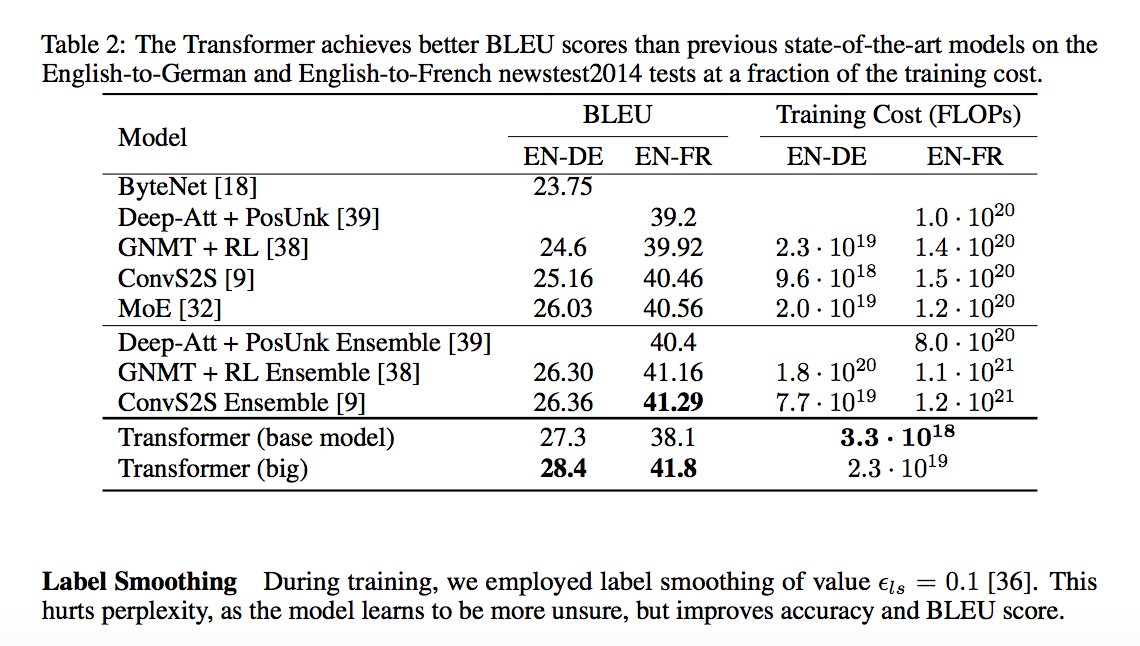#### 4.4.2 model variations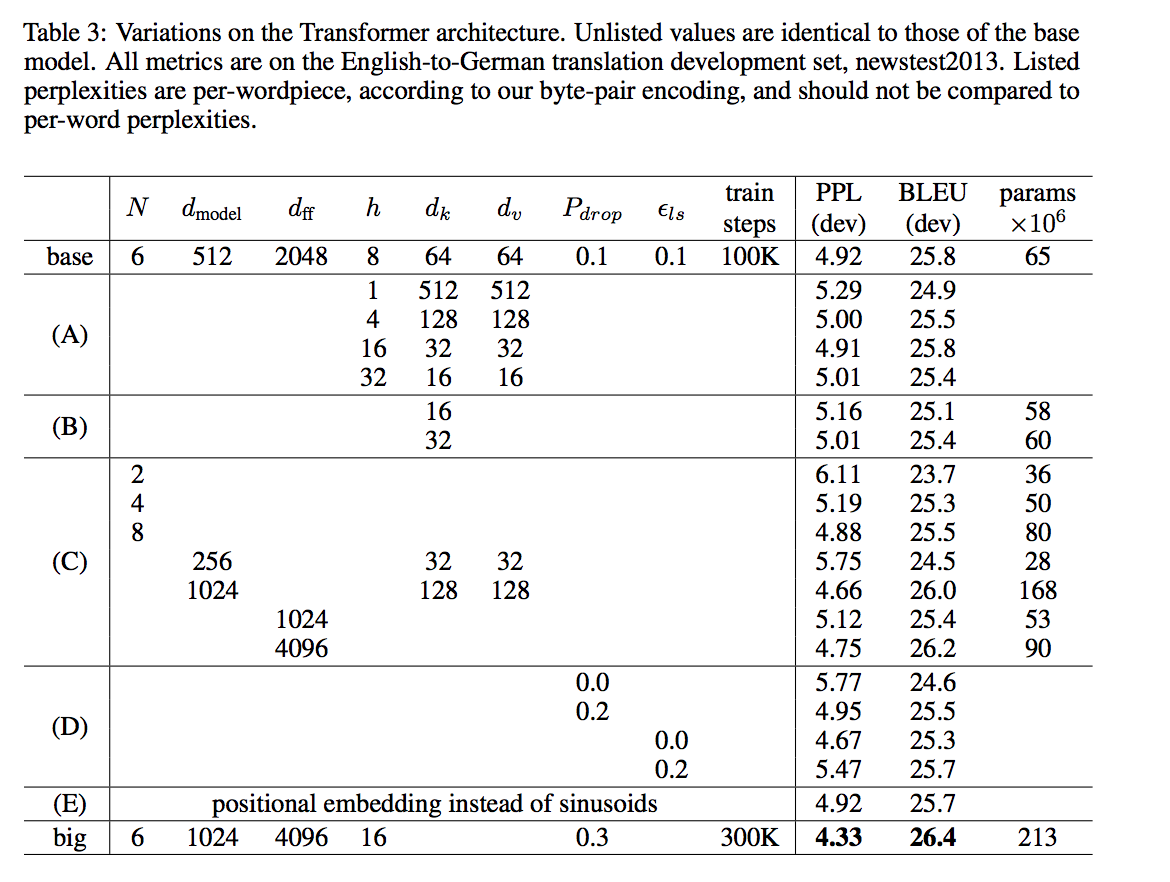#### 4.4.3 English Constituency Parsing

Constituency: 选区；选区的选民；支持者；主顾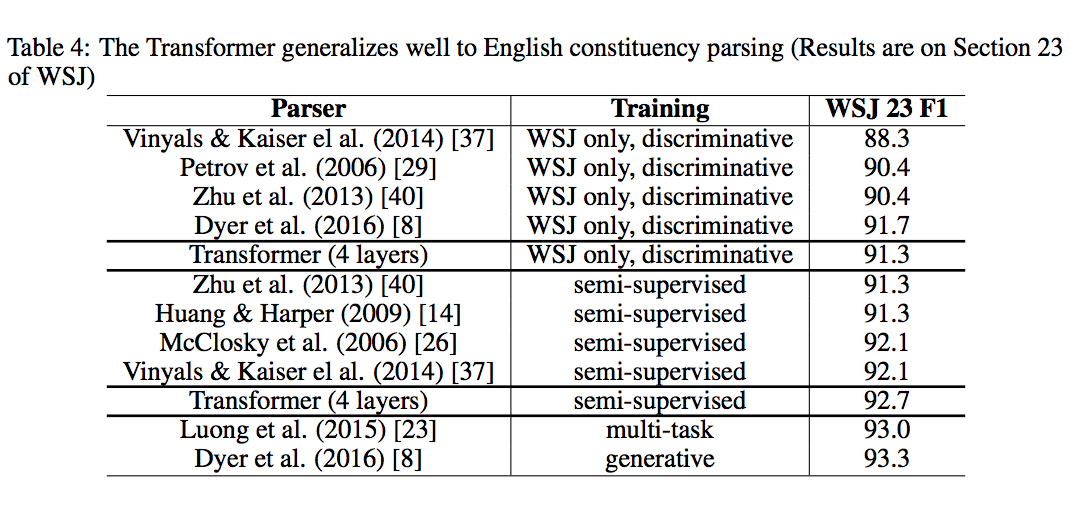## 6. 实践

• 安装cuda 8.0
• 安装cuda 8.0所需的对应cudnn版本（t2t要求cudnn5.0 for cuda 8.0）

# Assumes tensorflow or tensorflow-gpu installed
pip install tensor2tensor

# Installs with tensorflow-gpu requirement
pip install tensor2tensor[tensorflow_gpu]

# Installs with tensorflow (cpu) requirement
pip install tensor2tensor[tensorflow]


t2t-trainer \
--generate_data \
--data_dir=~/t2t_data \
--output_dir=~/t2t_train/mnist \
--problem=image_mnist \
--model=shake_shake \
--hparams_set=shake_shake_quick \
--train_steps=1000 \
--eval_steps=100


## 7. 代码解析

### 7.1 原版（tensor2tensor）

https://github.com/tensorflow/tensor2tensor

### 7.2 单纯transformer

https://github.com/Kyubyong/transformer

## 8. 改进

• 将 Transformer 中的 Multi-Head Attention 替换为多个自注意力分支，而模型会在训练阶段中将学习结合这些分支注意力模块。
• 采用了一种编码相对位置的表征以扩展自注意力机制，并令模型能更好地理解序列元素间的相对距离

## 9. 自己的小结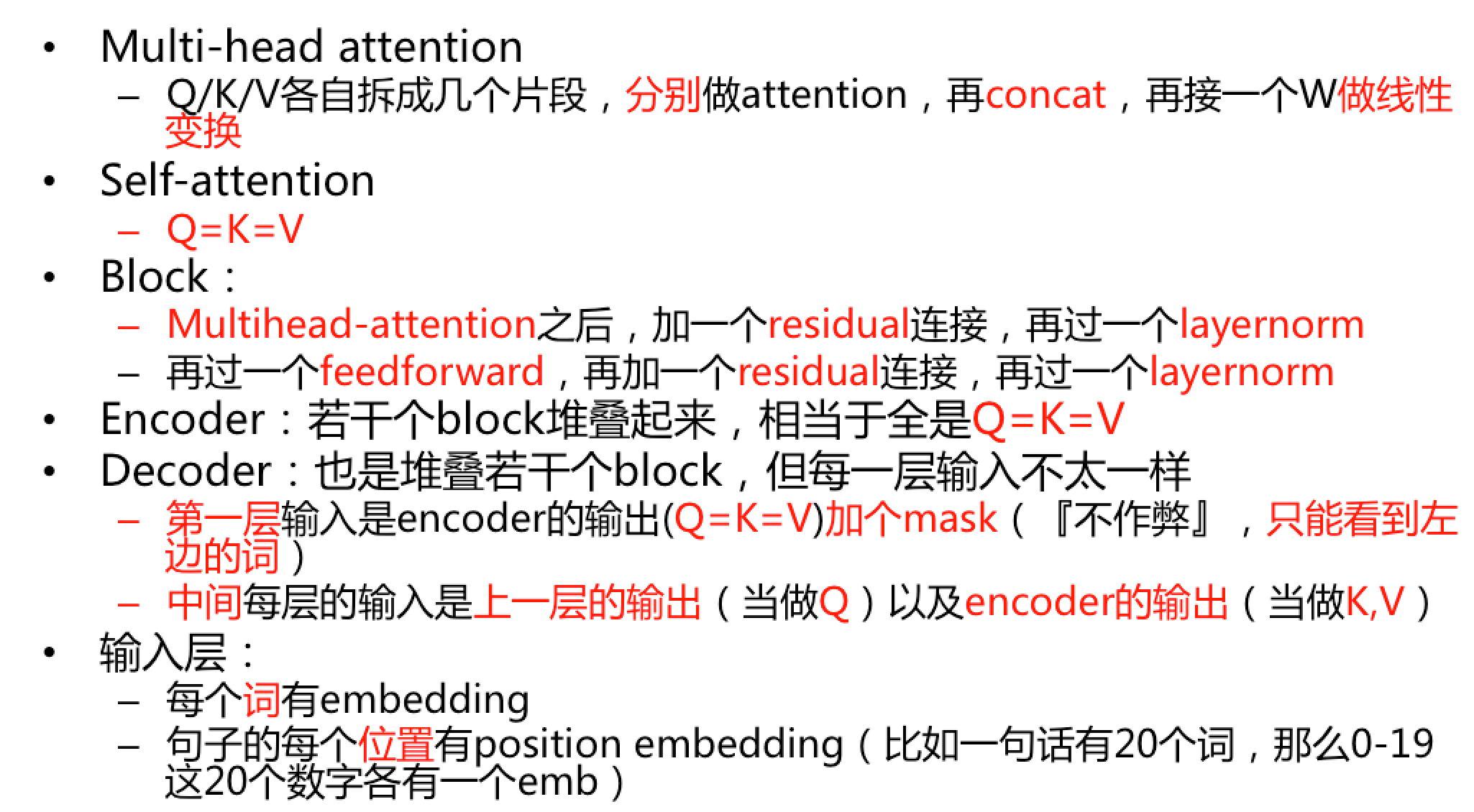comment here..